# Medication Temperature Monitoring System

Monitors the temperature in the cooling chambers of tablet storage, notifies anomaly(if detected) and helps maintain the required limits.

IntermediateFull instructions provided109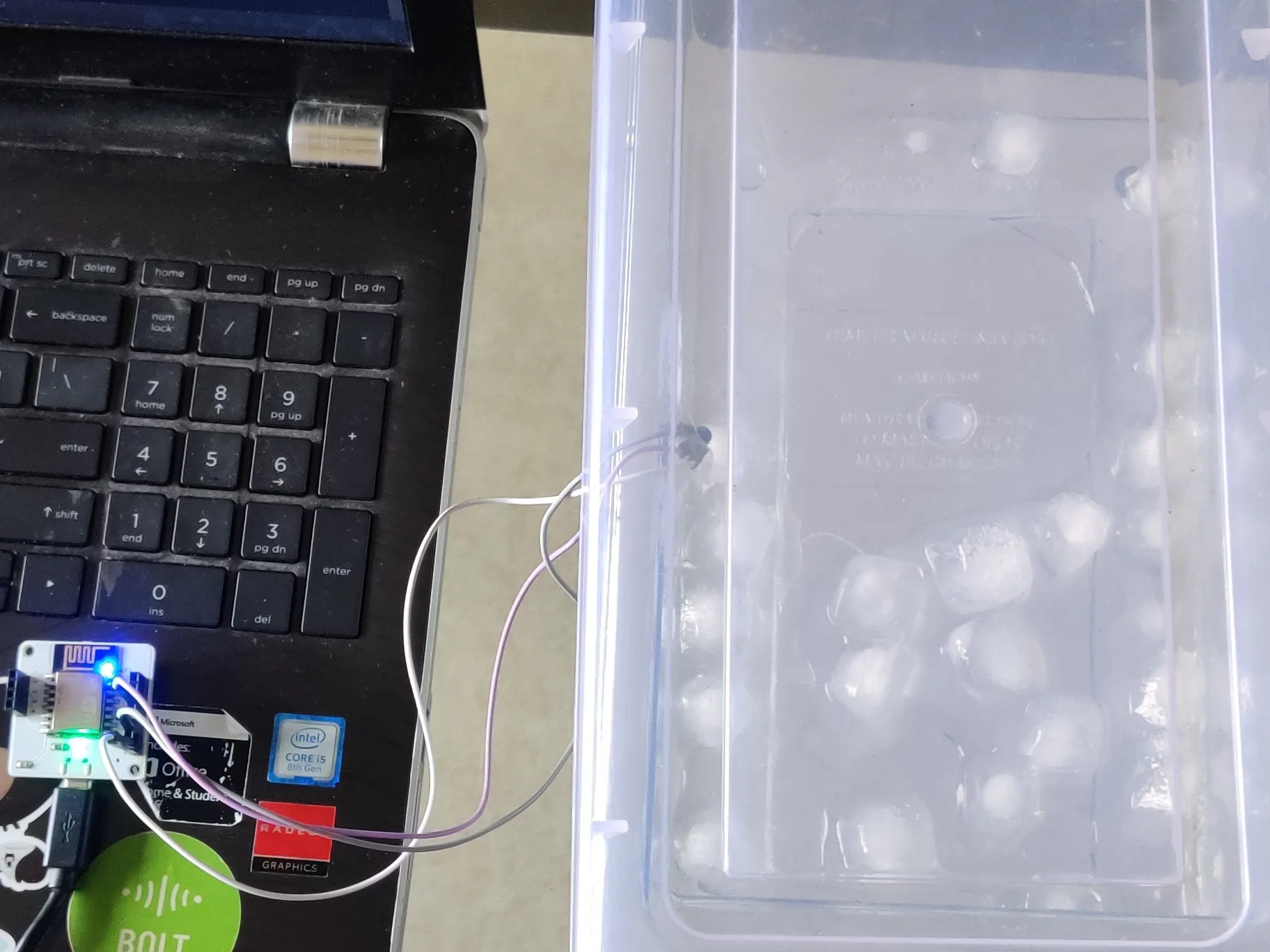## Things used in this project

### Hardware components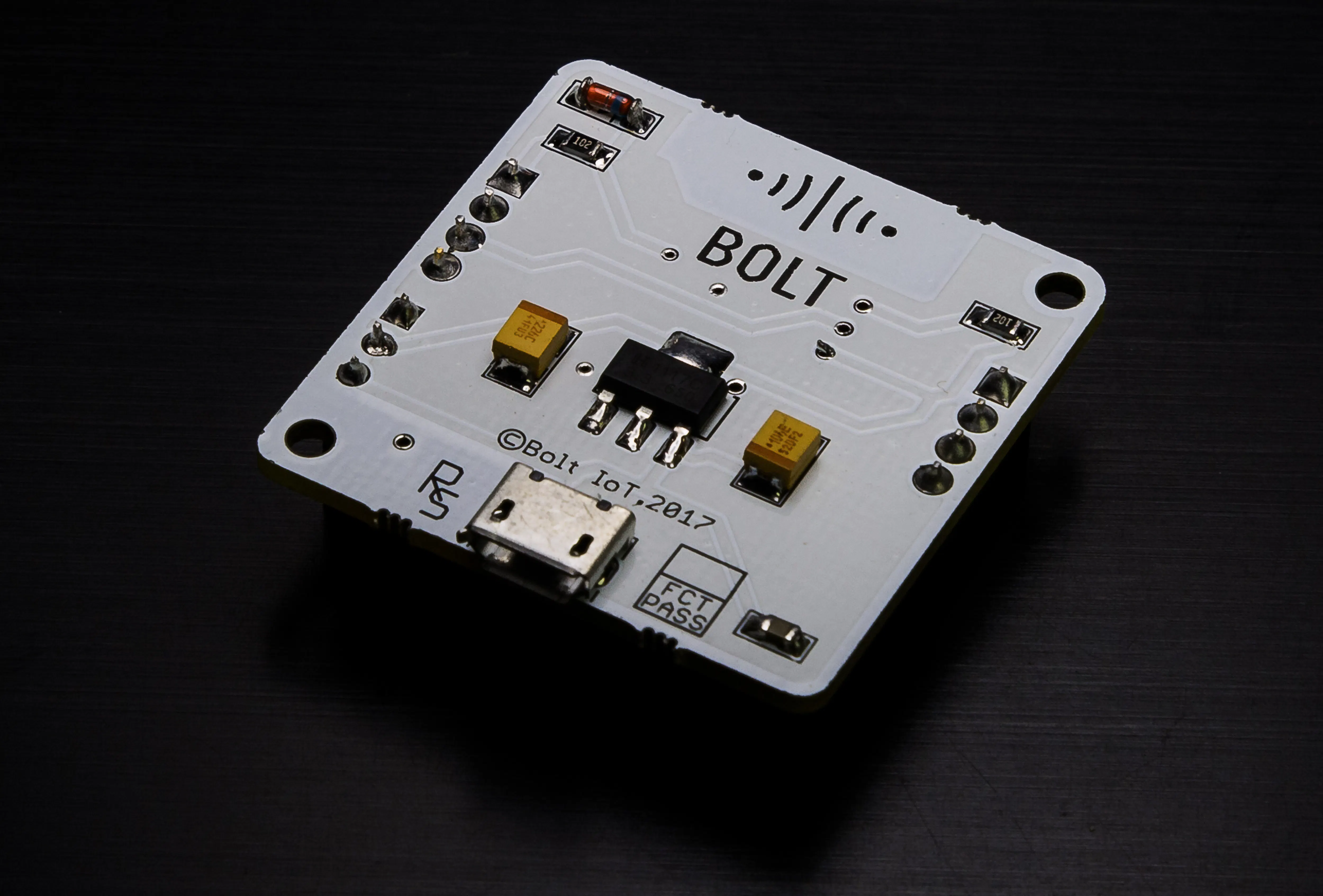Bolt IoT Bolt WiFi Module
×1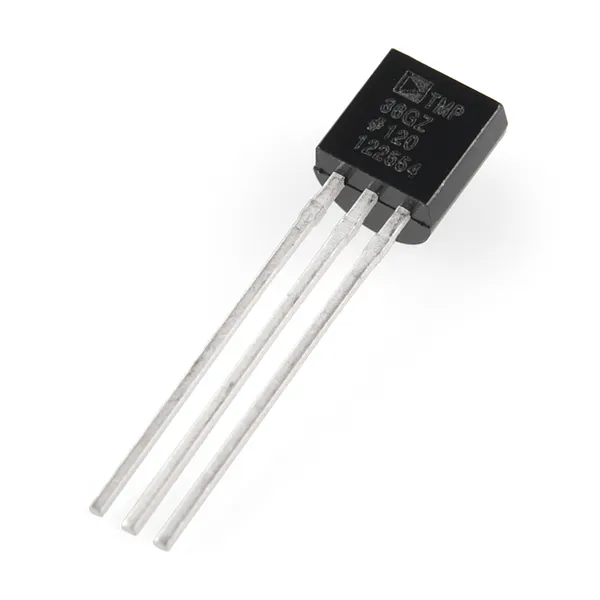Temperature Sensor
×1Jumper wires (generic)
×1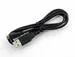USB-A to Micro-USB Cable
×1

### Software apps and online servicesBolt IoT Bolt Cloud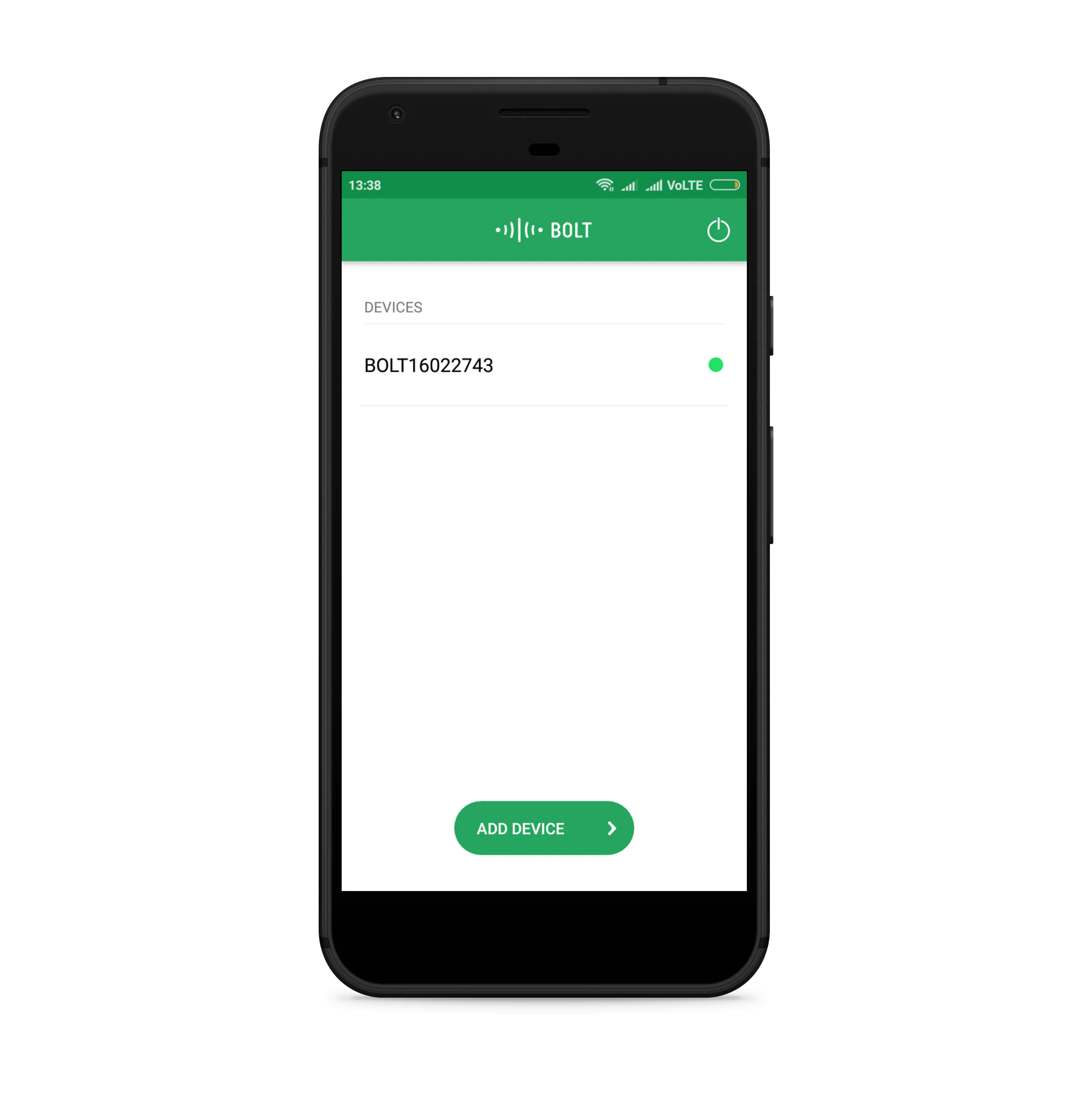Bolt IoT Android App
 Digital ocean
 Putty
 Mailgun

## Schematics

### Schematic diagram

Bolt wifi module to LM35 connection.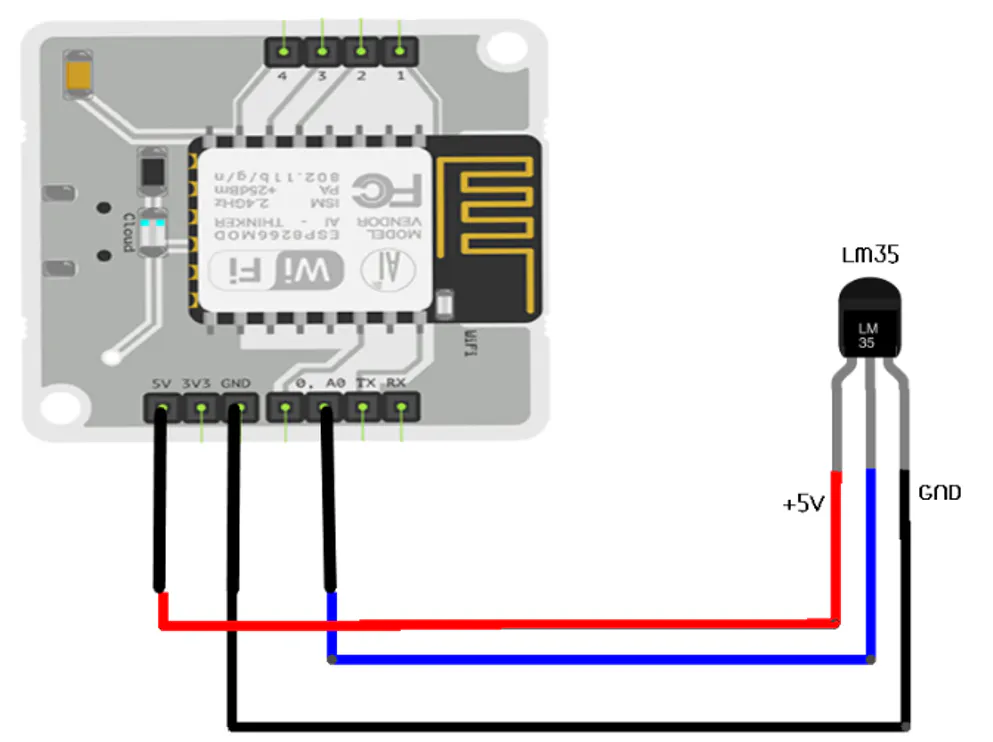## Code

### Sending Mail through Mailgun

Python
Stage-2
```import email_conf   #import the configuration file
from boltiot import Email, Bolt   #import the boltiot module and email from the Bolt python library
import json, time   #import various python libraries

minimum_limit = 277 #the minimum threshold of temperature
maximum_limit = 351 #the maximum threshold of temperature

mybolt = Bolt(email_conf.API_KEY, email_conf.DEVICE_ID)    #To identify your bolt device
mailer = Email(email_conf.MAILGUN_API_KEY, email_conf.SANDBOX_URL, email_conf.SENDER_EMAIL, email_conf.RECIPIENT_EMAIL)  # For sending mails through the mailgun account

while True:
response = mybolt.analogRead('A0')  #fetch the sensor value
data = json.loads(response)    #Retrieve the input data in json format
print ("Sensor value is: " + str(data['value']))
try:
sensor_value = int(data['value'])
#Check if it is in the specified range
if sensor_value > maximum_limit or sensor_value < minimum_limittry:
print("Making request to Mailgun to send an email")
response = mailer.send_email("Alert", "The Current temperature sensor value is " +str(sensor_value))
print("Response received from Mailgun is: " + str(response_text['message']))
except Exception as e:
print ("Error occured: Below are the details")
print (e)
time.sleep(10)
```

### Implementing Z-score Analysis

Python
Stage-3
```import email_conf   #import the configuration file
from boltiot import Email, Bolt   #import the boltiot module from the Bolt python library
import json, time, math, statistics #import various python libraries

#//---------FUNCTION TO COMPUTE BOUNDS OR Z SCORE ANALYSIS------------//
def compute_bounds(history_data,frame_size,factor):
#//Function to compute bounds
if len(history_data)<frame_size :
return None

if len(history_data)>frame_size :
del history_data[0:len(history_data)-frame_size]
Mn=statistics.mean(history_data)
Variance=0
for data in history_data :
Variance += math.pow((data-Mn),2)
Zn = factor * math.sqrt(Variance / frame_size)
High_bound = history_data[frame_size-1]+Zn
Low_bound = history_data[frame_size-1]-Zn
return [High_bound,Low_bound]  # //Returns Low Bound and High Bound

mybolt = Bolt(email_conf.API_KEY, email_conf.DEVICE_ID) # //To identify your bolt device
mailer = Email(email_conf.MAILGUN_API_KEY, email_conf.SANDBOX_URL, email_conf.SENDER_EMAIL, email_conf.RECIPIENT_EMAIL)    # //To identify Mailgun account
history_data=[]    # //Array of input values from LM35
while True:
data = json.loads(response) # //Retrieve the input data in json format
if data['success'] != 1:
print("There was an error while retriving the data.")
print("This is the error:"+data['value'])
time.sleep(10)
continue

print ("This is the value "+data['value'])
sensor_value=0
try:
sensor_value = int(data['value']) # //store current input value in variable
except e:
print("There was an error while parsing the response: ",e)
continue
#//----------------PERFORMING Z SCORE ANALYSIS--------------------------//
bound = compute_bounds(history_data,email_conf.FRAME_SIZE,email_conf.MUL_FACTOR)
if not bound:
required_data_count=email_conf.FRAME_SIZE-len(history_data)
print("Not enough data to compute Z-score. Need ",required_data_count," more data points")
history_data.append(int(data['value']))
time.sleep(10)
continue
try:
if sensor_value > bound :  # //If input crosses upper bound
print ("The temperature has increased suddenly. Sending an E-mail.")
response = mailer.send_email("Alert", "The temperature has suddenly increased! ")
print("This is the response ",response)
elif sensor_value < bound:   # //If input crosses lower bound
print ("The light level decreased suddenly. Sending an Email.")
response = mailer.send_email("Alert", "The temperature has suddenly decreased! ")
print("This is the response ",response)
history_data.append(sensor_value);   # //Append each new input to array history_data[]
except Exception as e:
print ("Error",e)
time.sleep(10)     # //Wait for 10 seconds
```

### Polynomial Regression

JavaScript
Stage 1
```setChartLibrary('google-chart')
setChartTitle('Polynomial Regression')
setChartType('predictionGraph')
setAxisName('time_stamp','temp')
mul(0.0977)
plotChart('time_stamp','temp')
```

## Credits

### VAISHNAVI PATIL

1 project • 0 followers
Thanks to Bolt IoT.